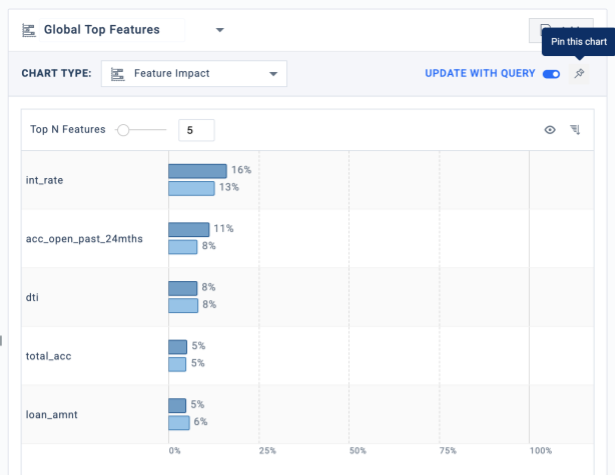# Analytics

UI Guide

## Interface

The Analyze tab has three parts:

1. Slice Query box (top-left) — Accepts a SQL query as input, allowing quick access to the slice.
2. Data table (bottom-left) — Lets you browse instances of data returned by the query.
3. Explanations column (right) — Allows you to view explanations for the slice and choose from a range of rich visualizations for different data insights.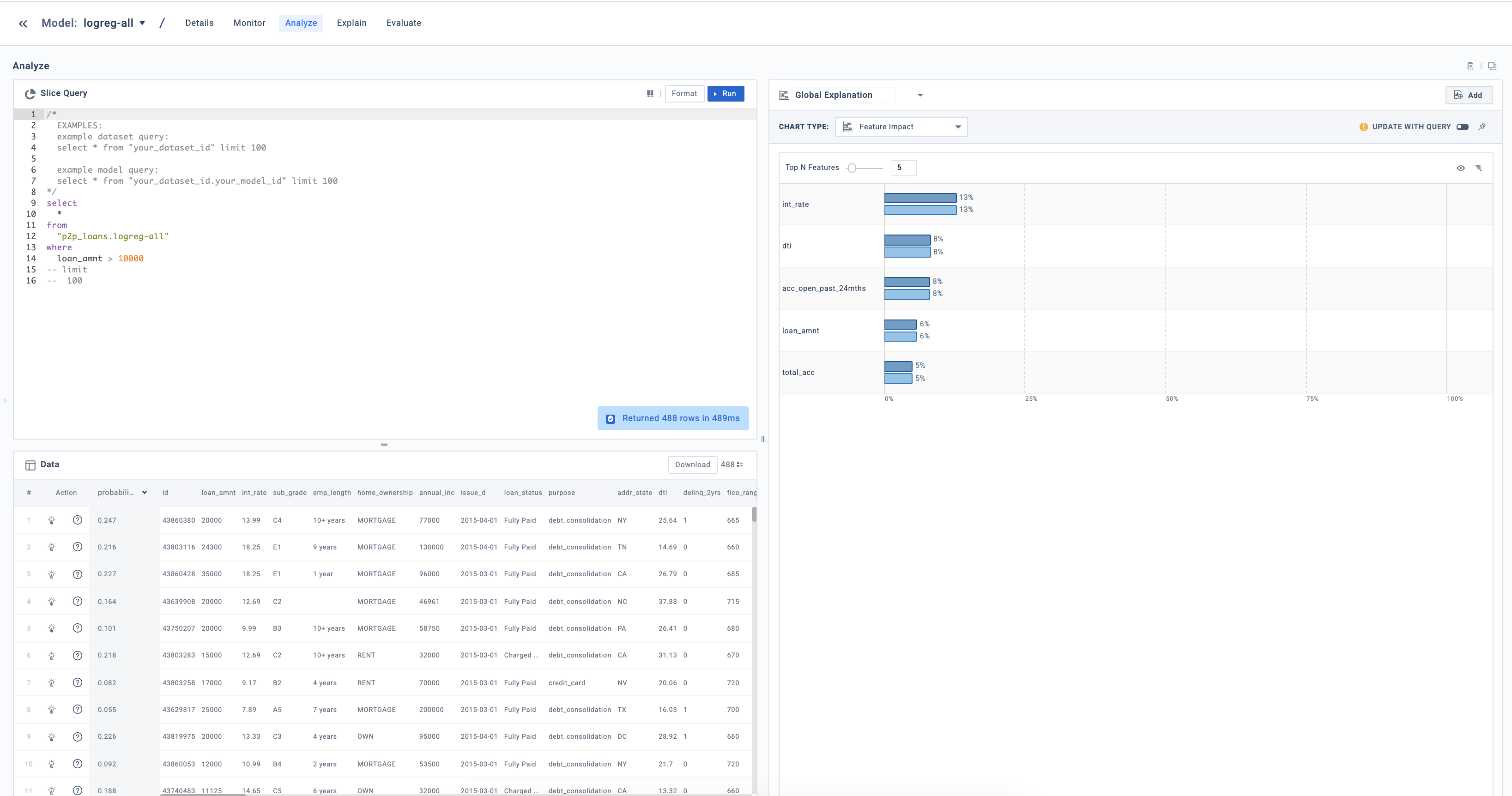Workflow

1. Write a SQL query in the Slice Query box and click Run.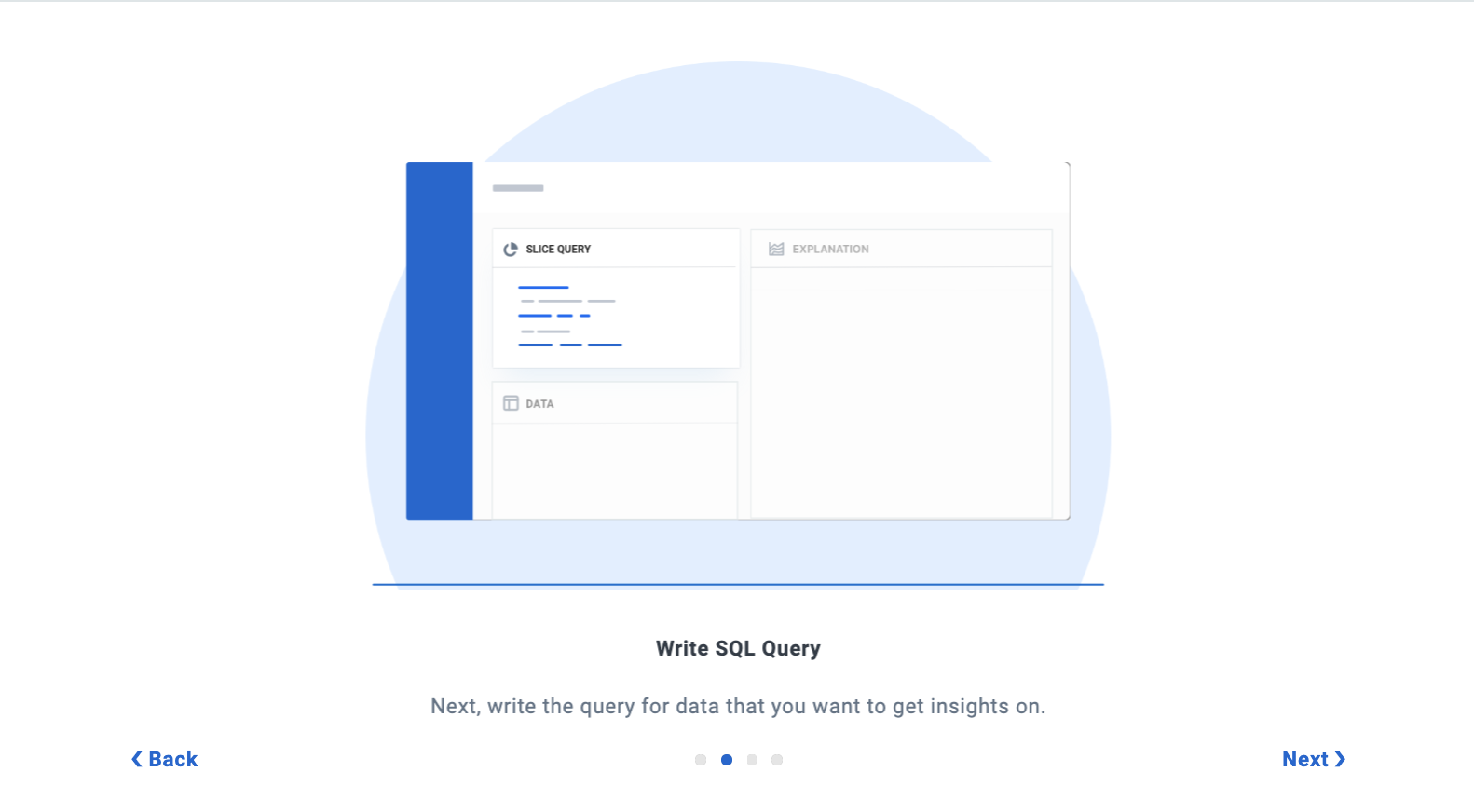1. View the data returned by the query in the Data table.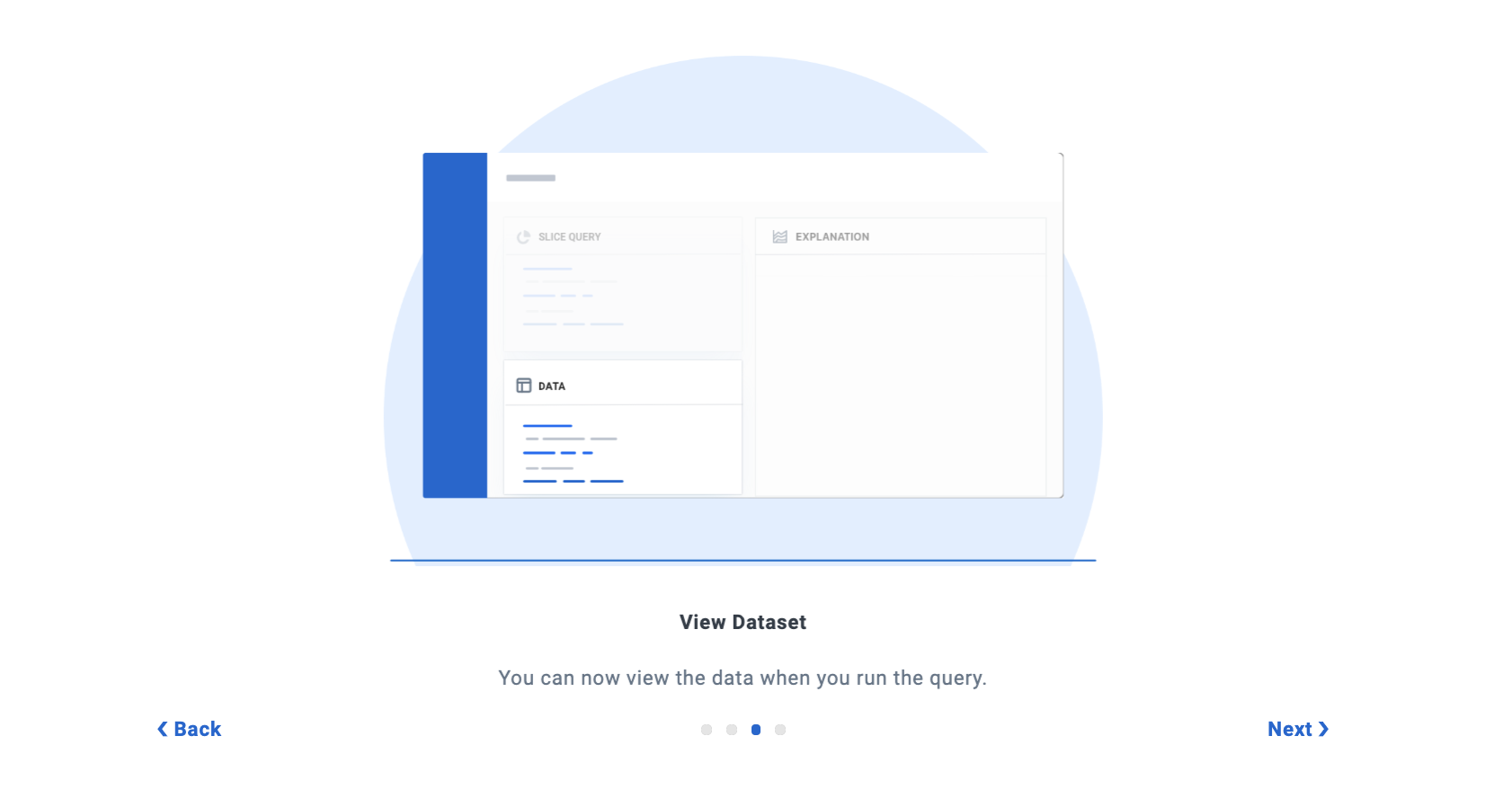1. Explore a variety of visualizations using the Explanations column on the right.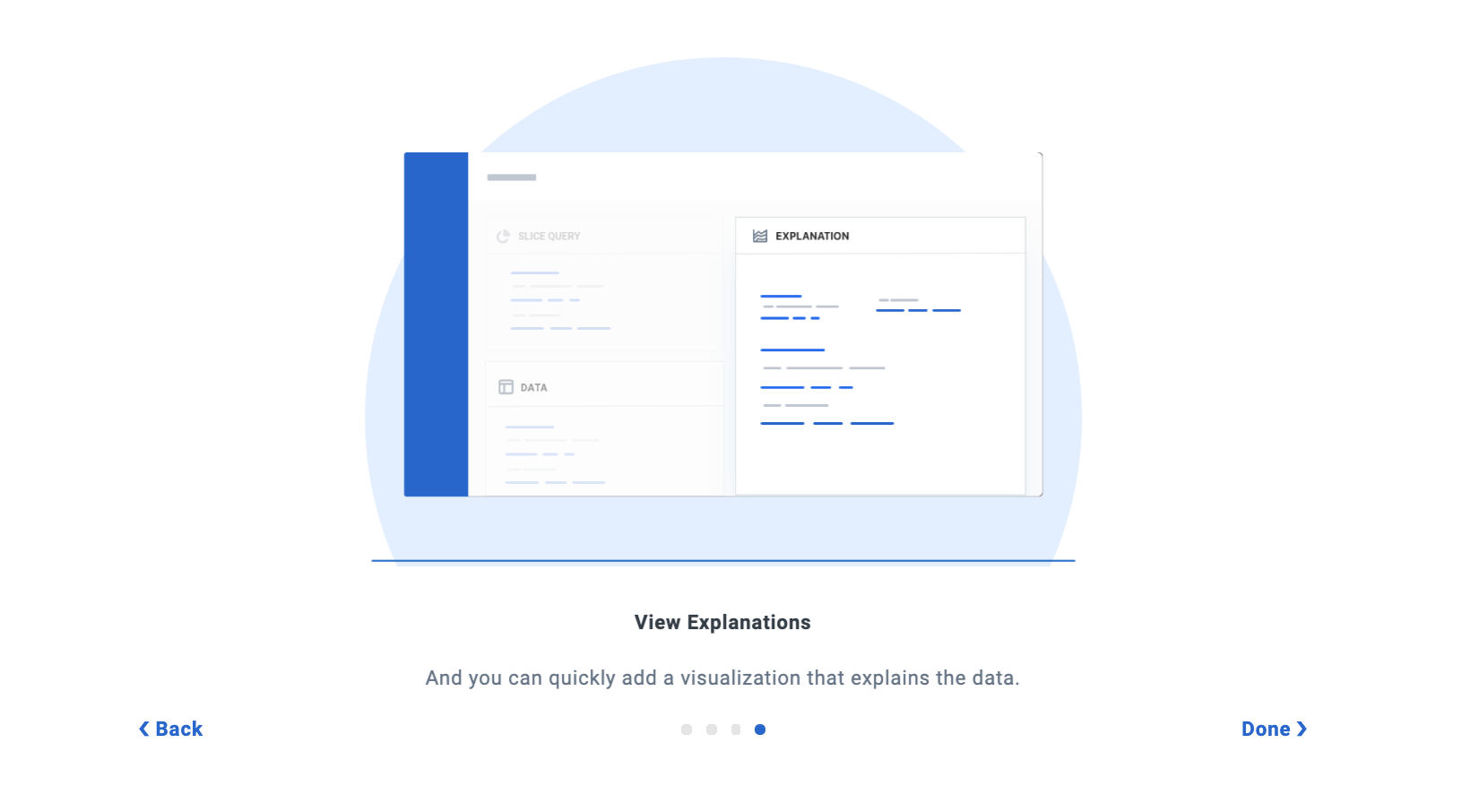## SQL Queries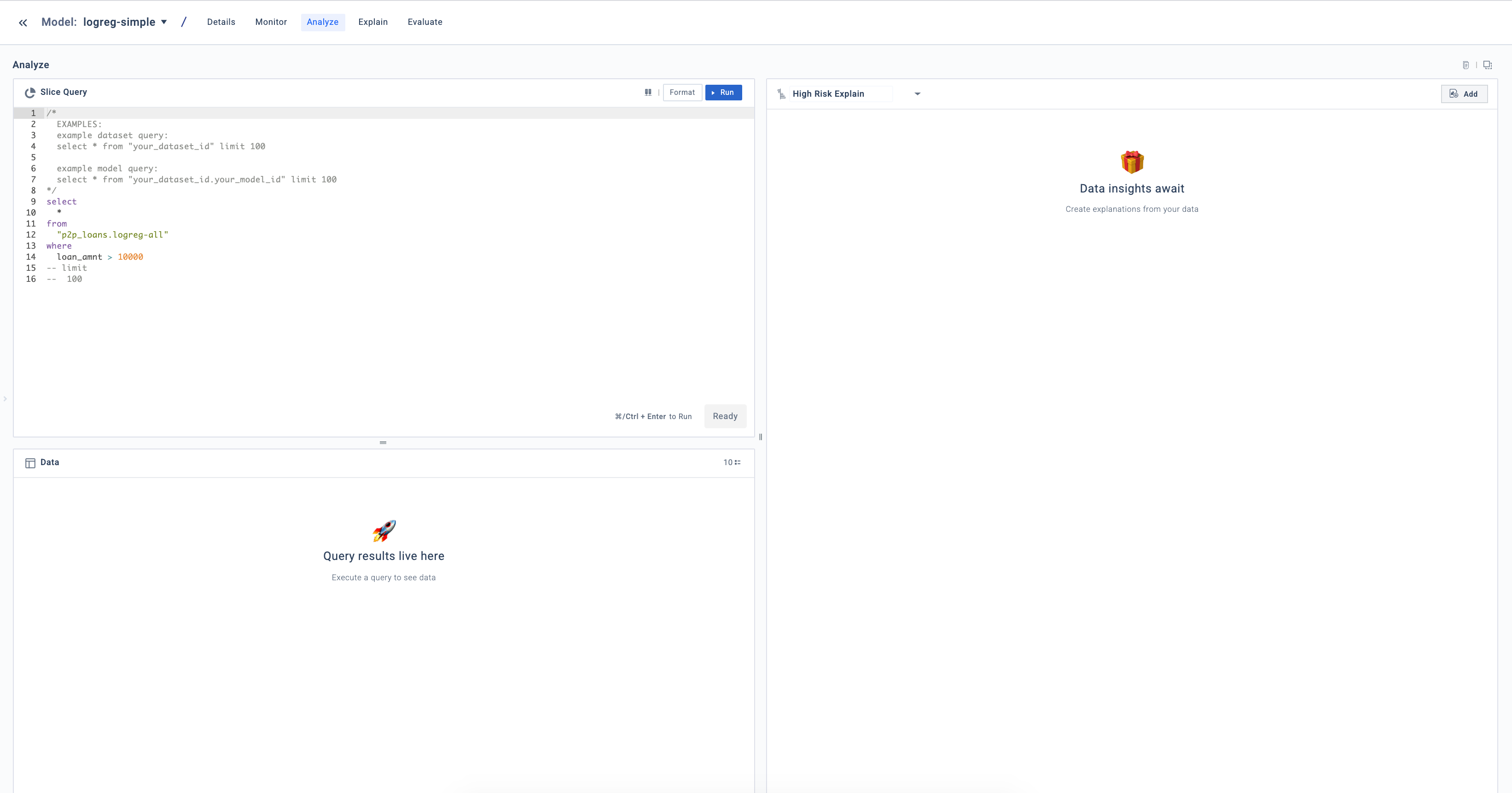The Slice Query box lets you:

1. Write a SQL query
2. Search and auto-complete field names (i.e. your dataset, the names of your inputs or outputs)
3. Run the SQL query

In the UI, you will see examples for different types of queries:

• Example query to analyze your dataset:
``````select * from "your_dataset_id" limit 100
``````
• Example query to analyze your model:
``````select * from "your_dataset_id.your_model_id" limit 100
``````
• Example query to analyze production traffic:
``````select * FROM production."your_model_id"
where fiddler_timestamp between '2020-10-20 00:00:00' AND '2020-10-20 12:00:00'limit 100
``````

🚧

Note

Only read-only SQL operations are supported. Slices are auto-detected based on your model, dataset, and query. Certain SQL operations like aggregations and joins might not result in a valid slice.

## Data

If the query successfully returns a slice, the results display in the Data table below the Slice Query box.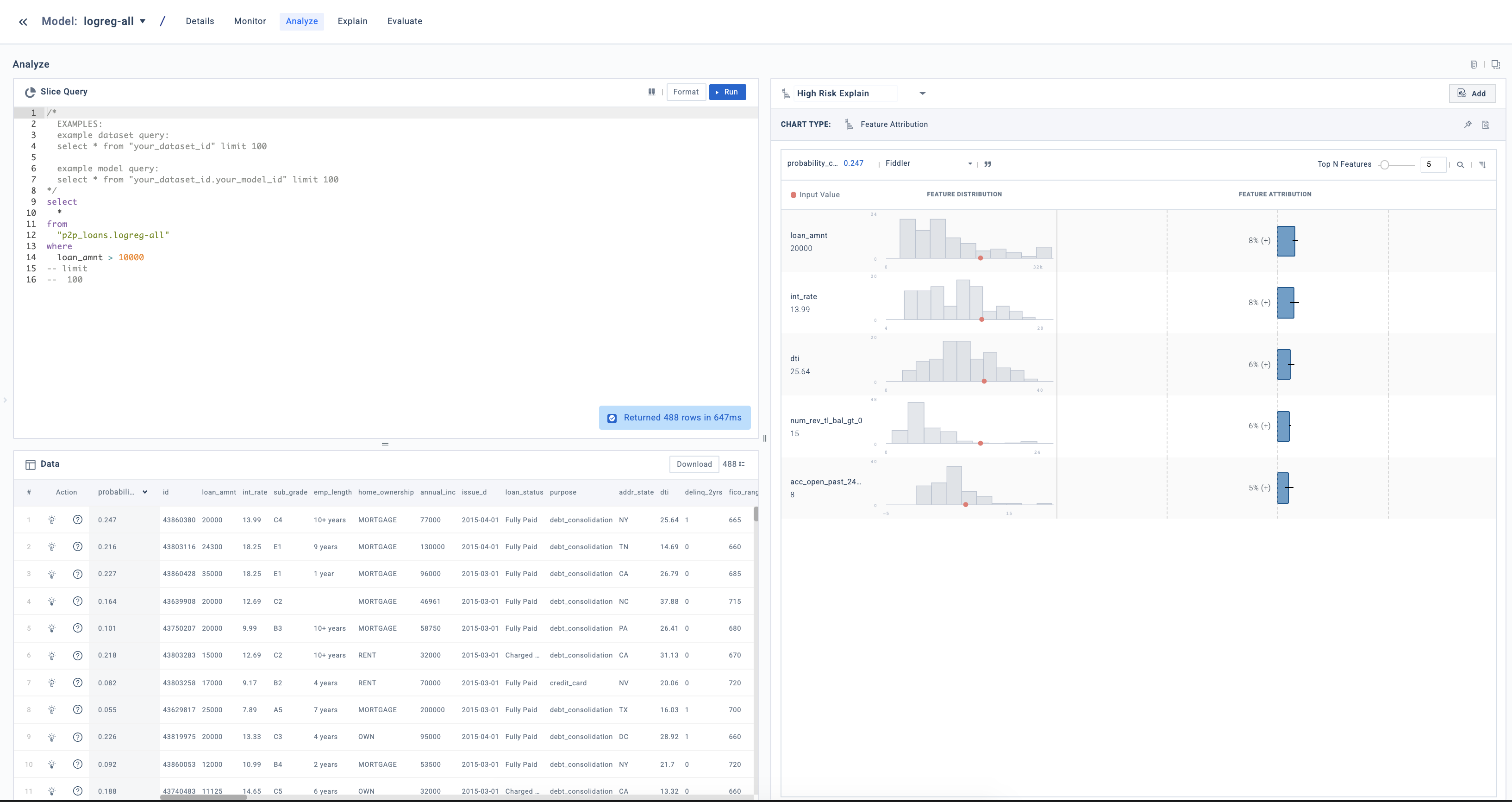You can view all data rows and their values or download the data as a CSV file to plug it into another system. By clicking on Explain (light bulb icon) in any row in the table, you can access explanations for that individual input (more on this in the next section).

## Explanations

The Analyze tab offers a variety of powerful visualizations to quickly let you analyze and explain slices of your dataset.

1. Feature Correlation — View the correlation between model inputs and/or outputs.
2. Feature Distribution — Visualize the distribution of an input or output.
3. Feature Impact — Understand the aggregate impact of model inputs to the output.
4. Partial Dependence Plot — Understand the aggregate impact of a single model input in its output.
5. Slice Evaluation — View the model metrics for a given slice.
6. Dataset Details — Analyze statistical qualities of the dataset.

You can also access the following point explanation methods by clicking on Explain (light bulb icon) for a given data point:

1. Point Overview — Get an overview of the model inputs responsible for a prediction.
2. Feature Attribution — Understand how responsible each model input is for the model output.
3. Feature Sensitivity – Understand how changes in the model’s input values will impact the model’s output.

📘

Info

## Feature Correlation

The feature correlation visualization plots a single variable against another variable. This plot helps identify any visual clusters that might be useful for further analysis. This visualization supports integer, float, and categorical variables.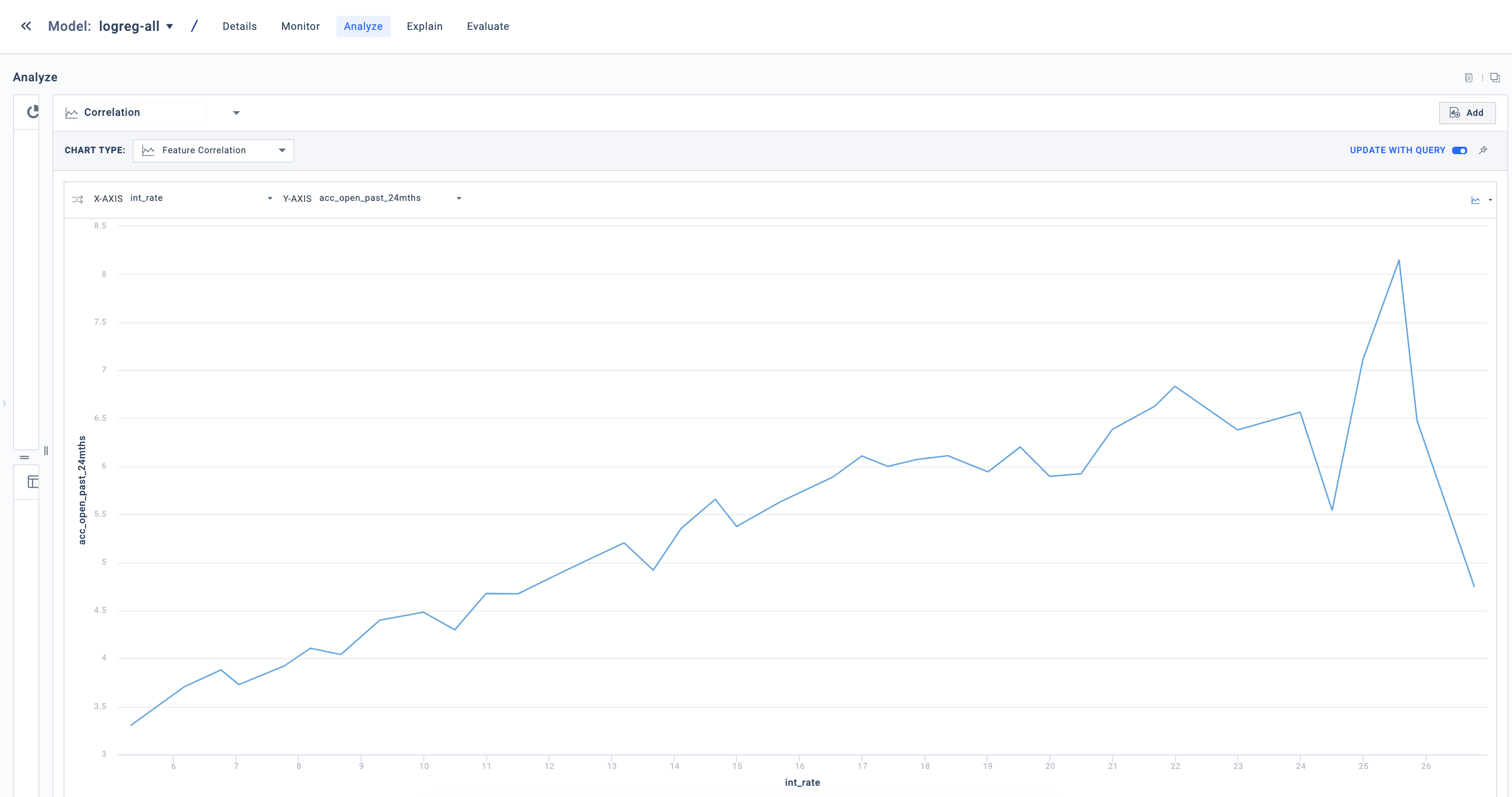## Feature Distribution

The feature distribution visualization is one of the most basic plots, used for viewing how the data is distributed for a particular variable. This plot helps surface any data abnormalities or data insights to help root-cause issues or drive further analysis.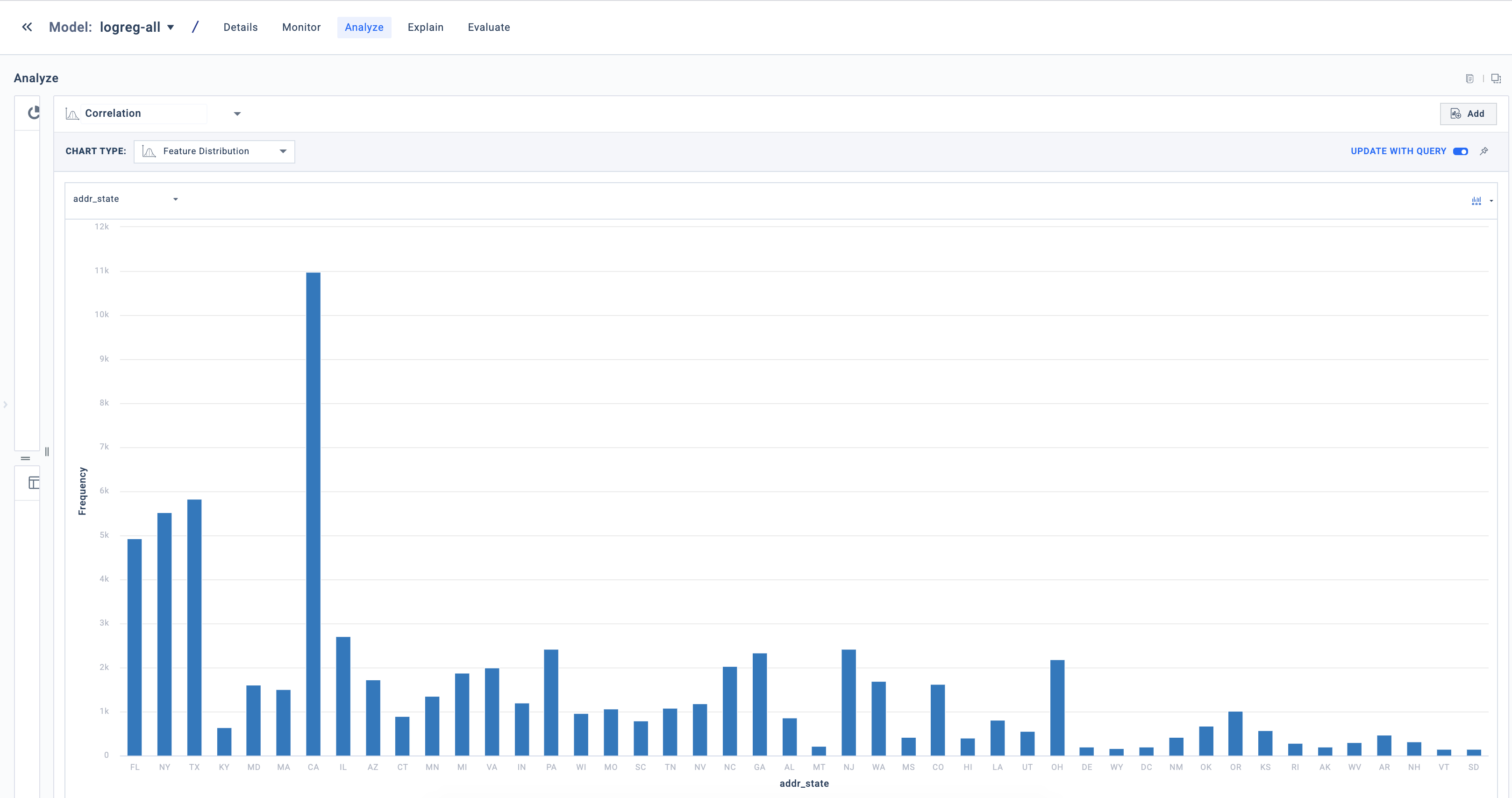## Feature Impact

This visualization provides the feature impact of the dataset (global explanation) or the selected slice (local explanation), showcasing the overall sensitivity of the model output to each feature (more on this in the Global Explainability section). We calculate Feature Impact by randomly intervening on every input using ablations and noting the average absolute change in the prediction.

A high impact suggests that the model’s behavior on a particular slice is sensitive to changes in feature values. Feature impact only provides the absolute impact of the input—not its directionality. Since positive and negative directionality can cancel out, we recommend using a Partial Dependence Plot (PDP) to understand how an input impacts the output in aggregate.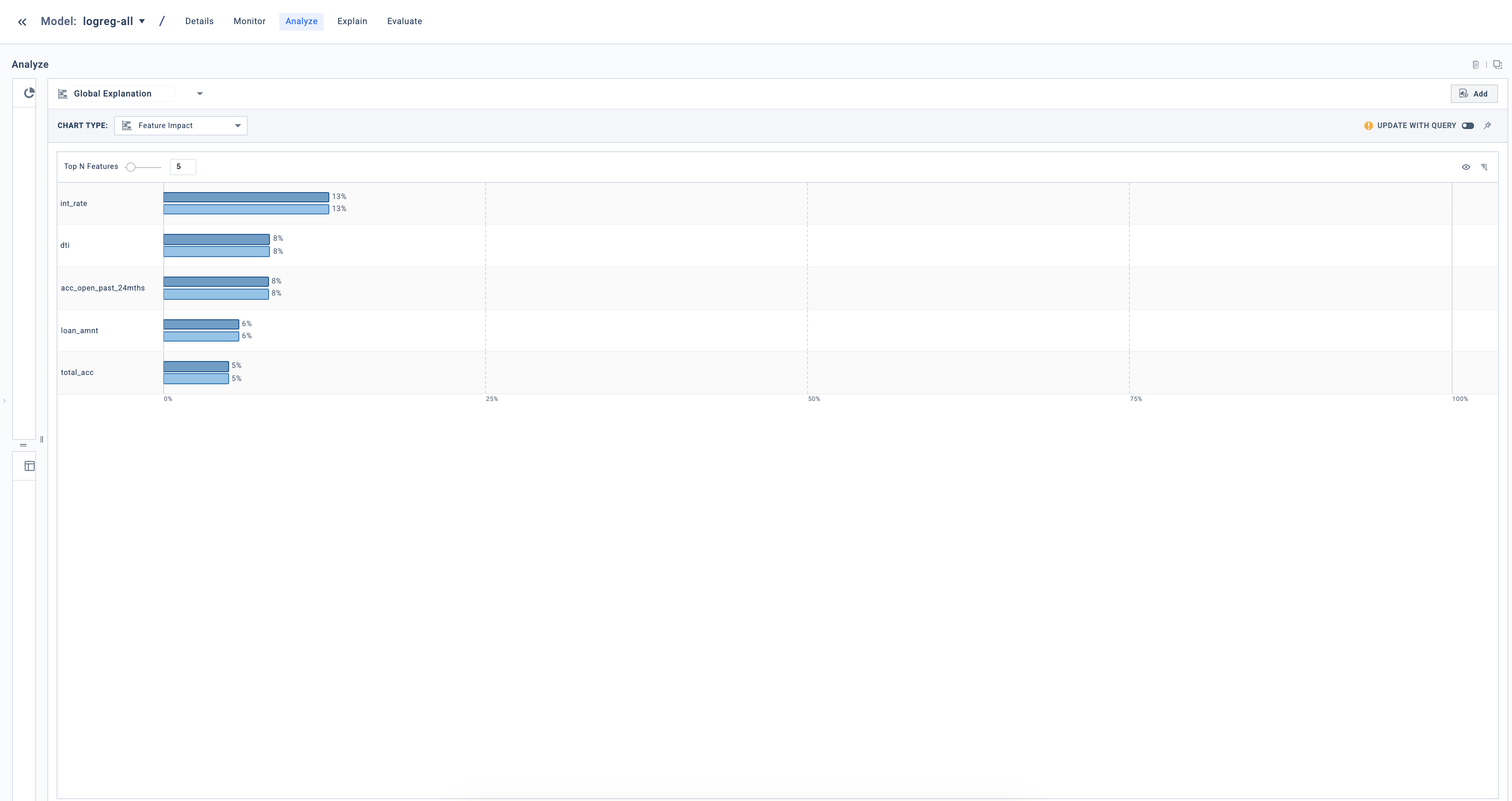## Partial Dependence Plot (PDP)

Partial dependence plots show the marginal effect of a selected model input on the model output. This plot helps understand whether the relationship between the input and the output is linear, monotonic, or more complex.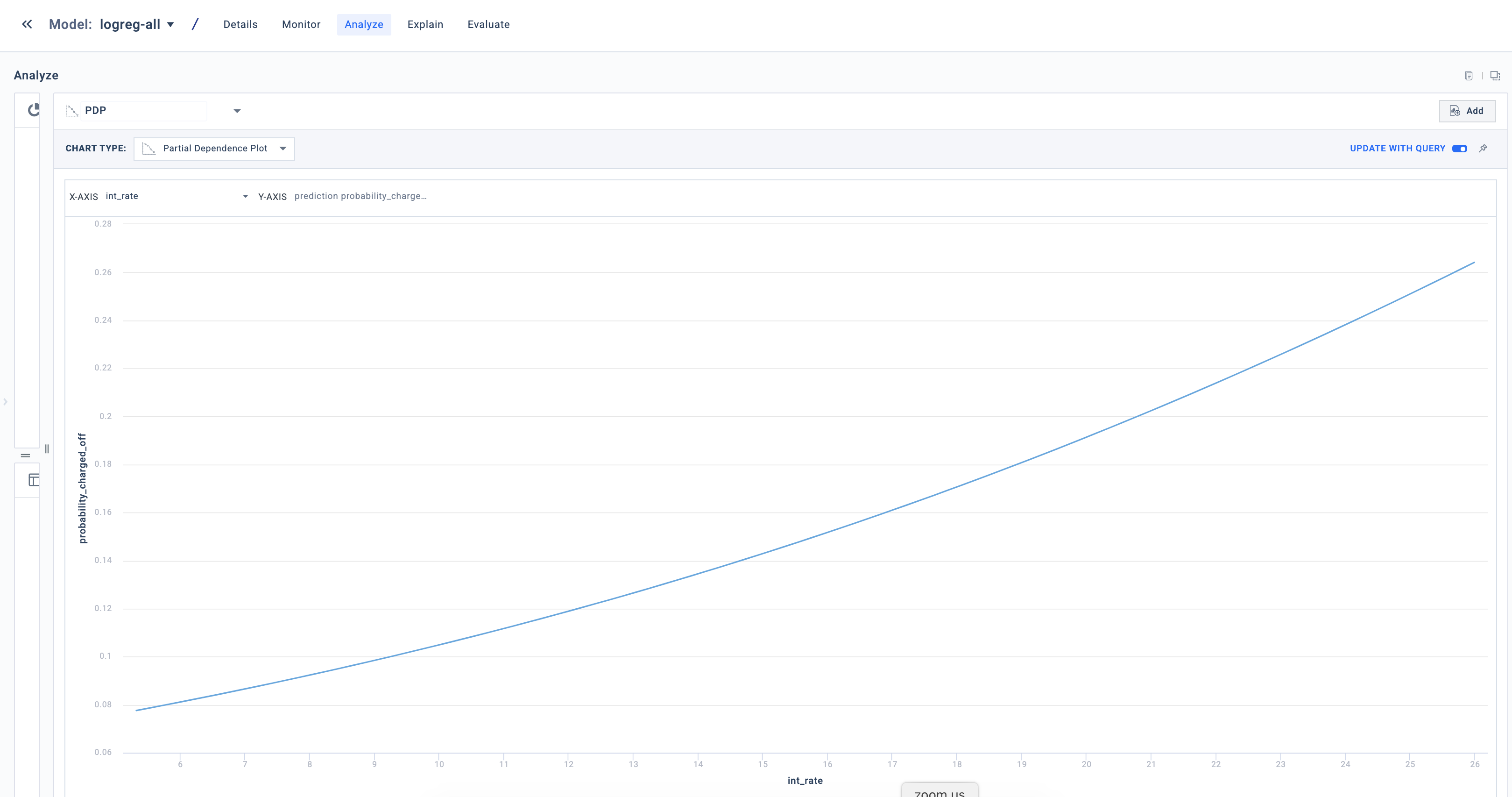## Slice Evaluation

The slice evaluation visualization gives you key model performance metrics and plots, which can be helpful to identify performance issues or model bias on protected classes. In addition to key metrics, you get a confusion matrix along with precision recall, ROC, and calibration plots. This visualization supports classification, regression, and multi-class models.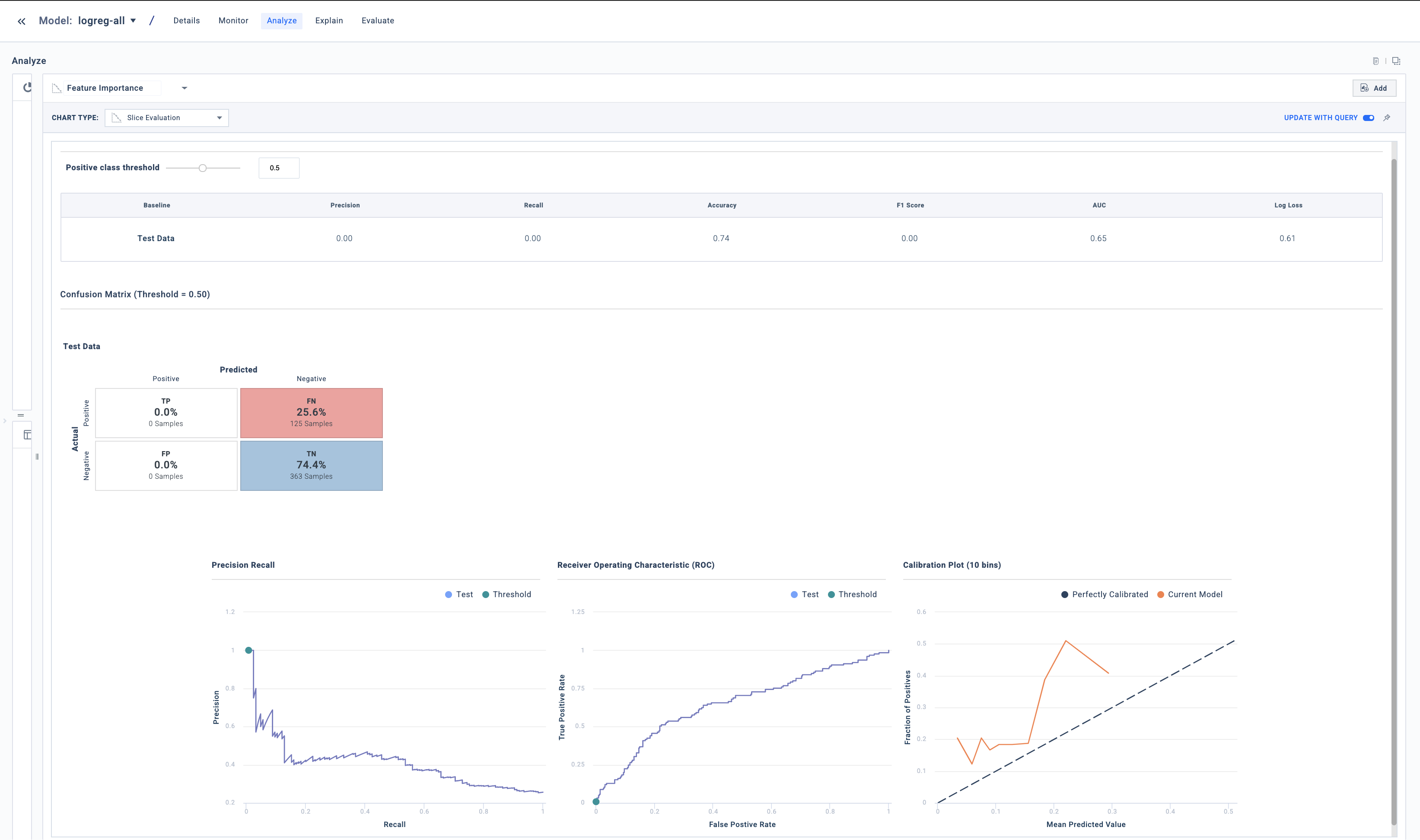## Dataset Details

This visualization provides statistical details of your dataset to help you understand the data’s distribution and correlations.

Select a target variable to see the dependence between that variable and the others, measured by mutual information (MI). A low MI is an indicator of low correlation between two variables, and can be used to decide if particular variables should be dropped from the model.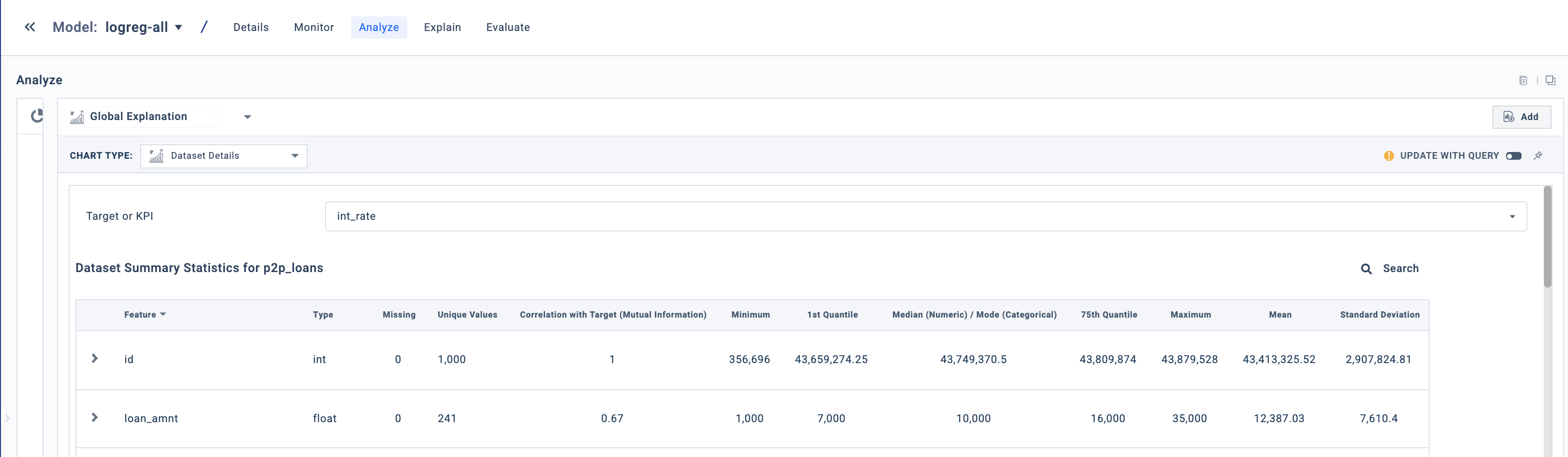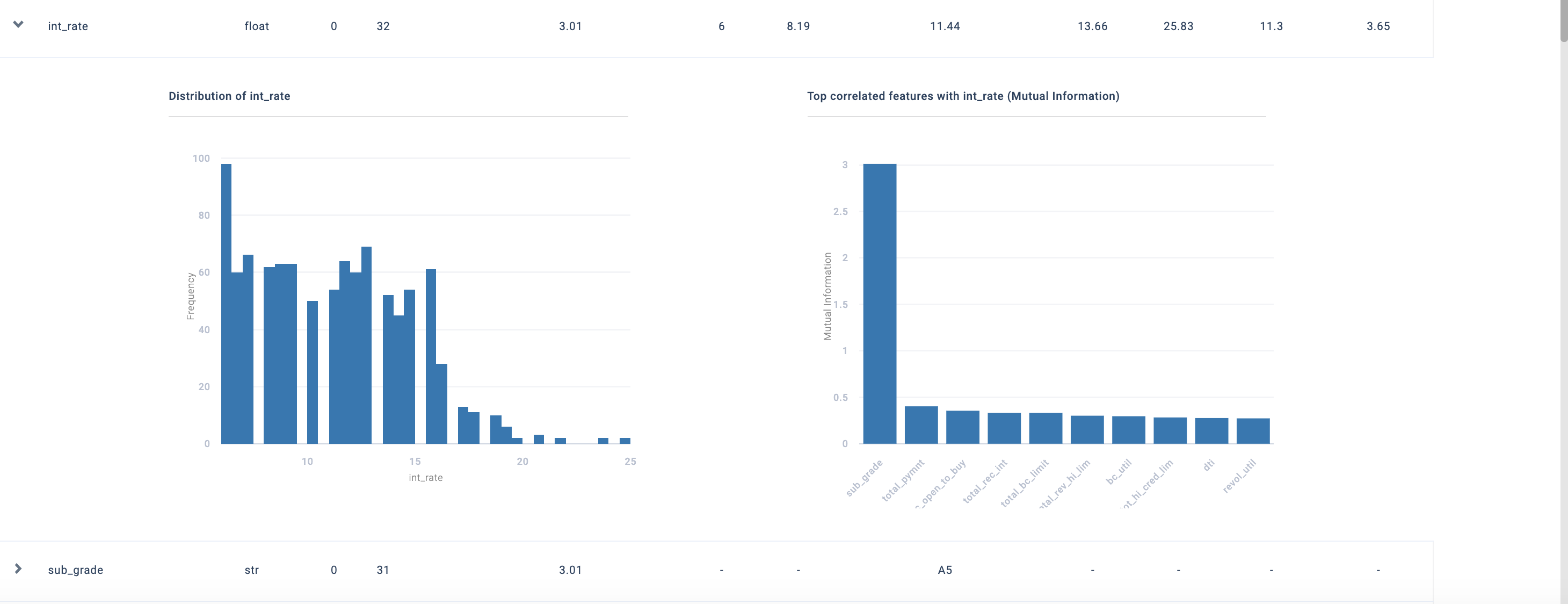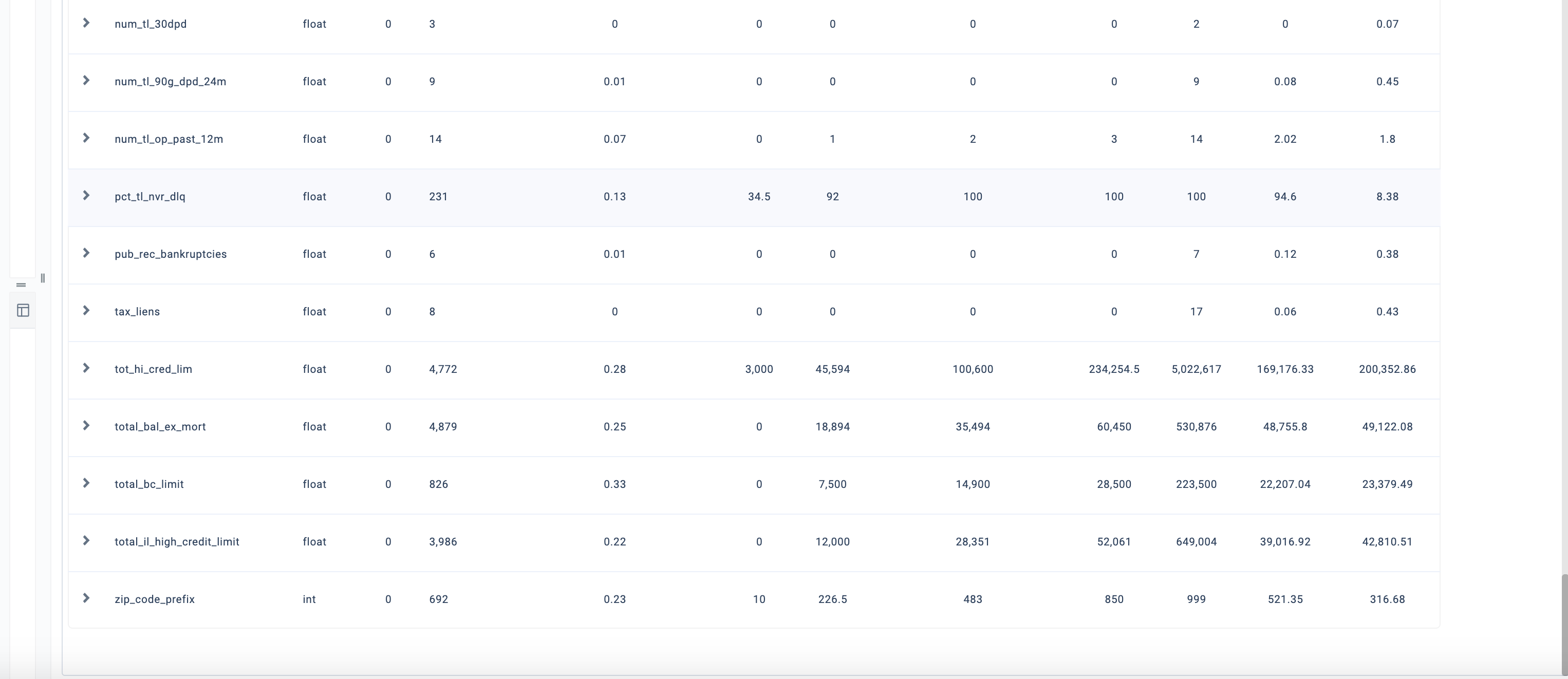## Point Overview

📘

Info

To view this visualization, click on Explain (light bulb icon) for any row in the Data table.

This visualization provides a human-readable overview of a point explanation.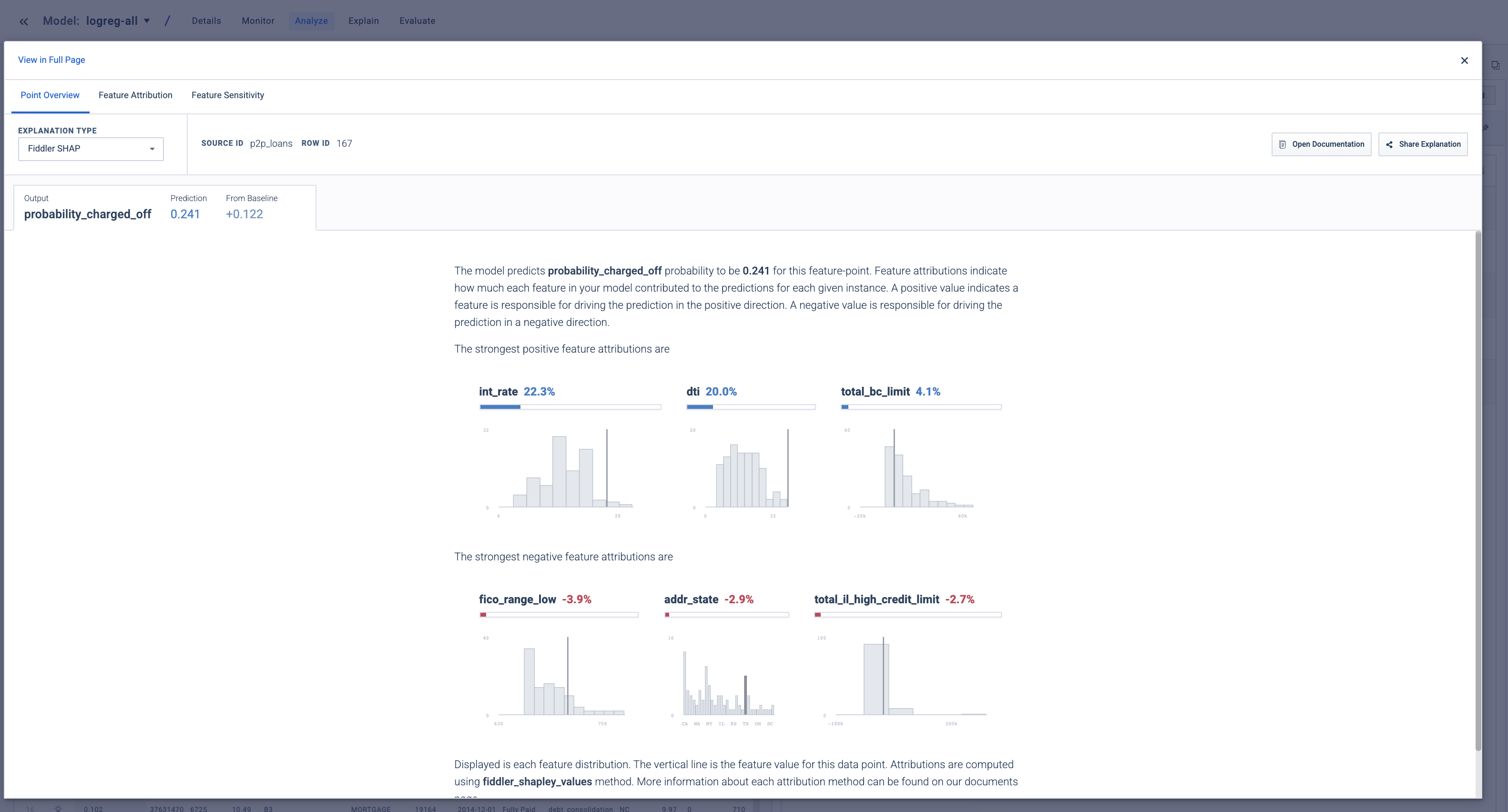📘

Info

To view this visualization, click on Explain (light bulb icon) for any row in the Data table.

Feature attributions can help you understand which model inputs were responsible for arriving at the model output for a particulat prediction.

When you want to check how the model is behaving for one prediction instance, use this visualization first.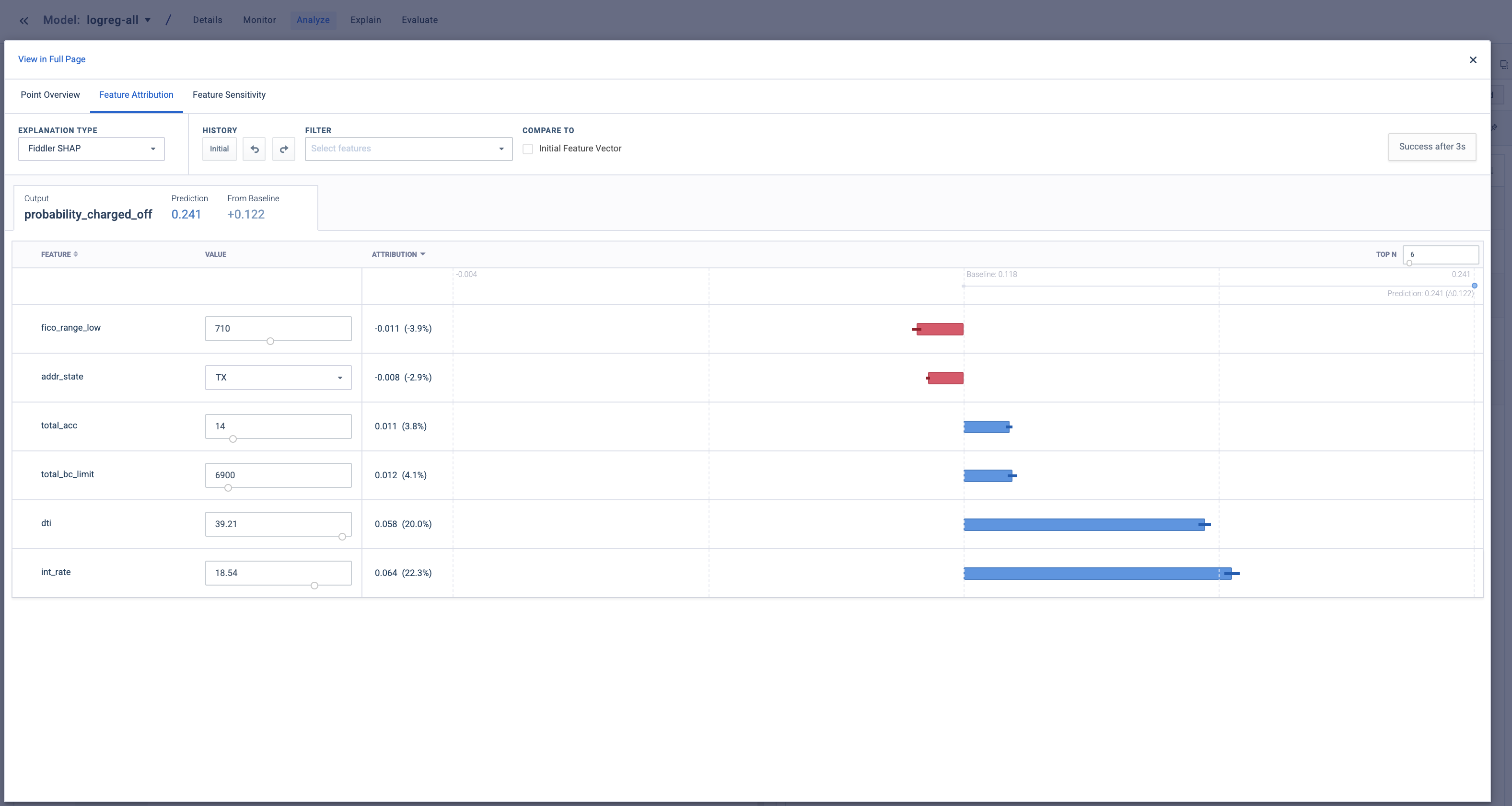## Feature Sensitivity

📘

Info

To view this visualization, click on Explain (light bulb icon) for any row in the Data table.

This visualization helps you understand how changes in the model’s input values could impact the model’s prediction for this instance.

ICE plots

On initial load, the visualization shows an Individual Conditional Expectation (ICE) plot for each model input.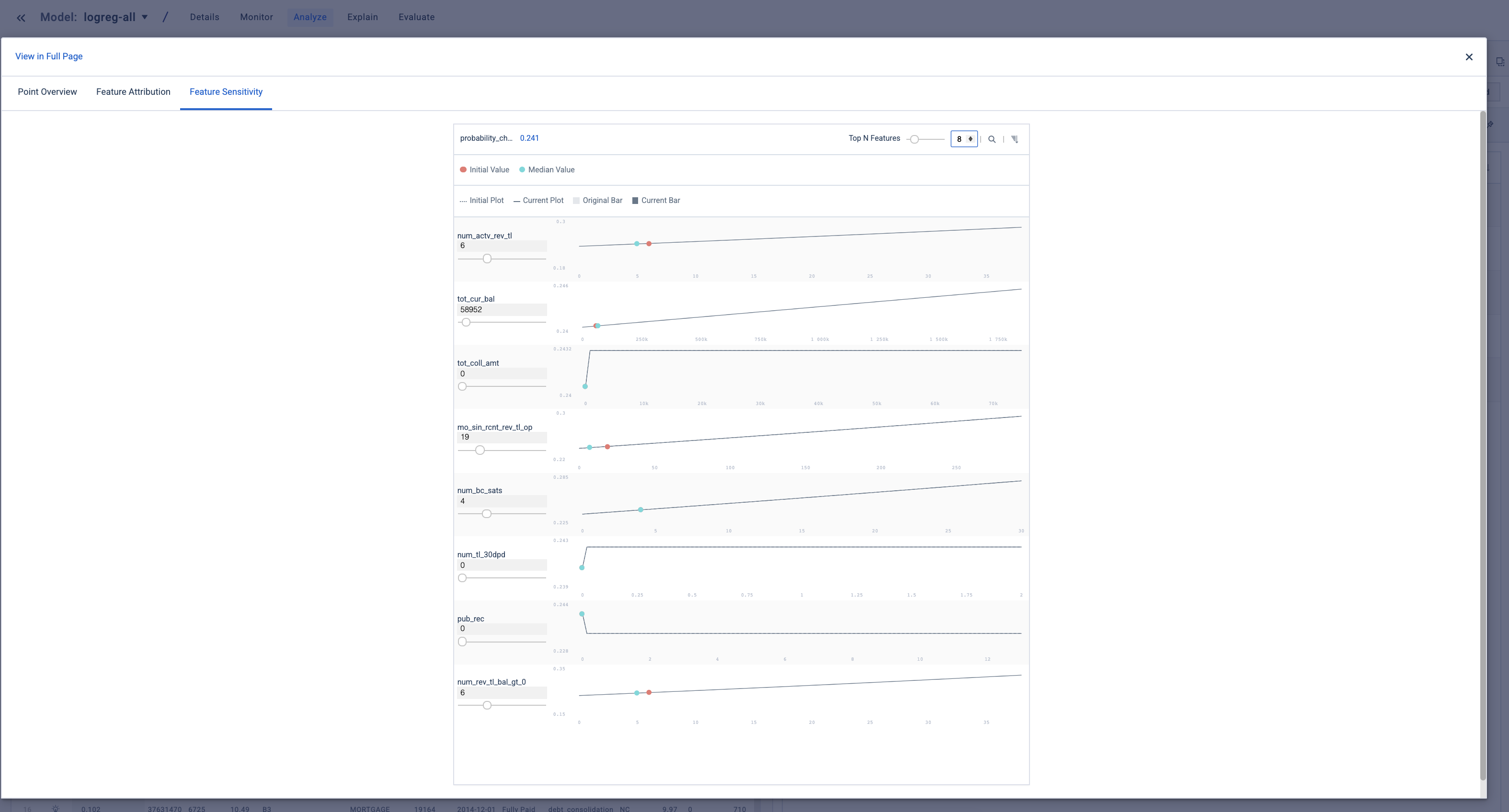ICE plots shows how the model prediction is affected by changes in an input for a particular instance. They’re computed by changing the value of an input—while keeping all other inputs constant—and plotting the resulting predictions.

Recall the partial dependence plots discussed earlier, which showed the average effect of the feature across the entire slice. In essence, the PDP is the average of all the ICE plots. The PDP can mask interactions at the instance level, which an ICE plot will capture.

You can update any input value to see its impact on the model output, and then view the updated ICE plots for the changed input values.

This is a powerful technique for performing counterfactual analysis of a model prediction. When you plot the updated ICE plots, you’ll see two lines (or sets of bars in the case of categorical inputs).

In the image below, the solid line is the original ICE plot, and the dotted line is the ICE plot using the updated input values. Comparing these two sets of plots can help you understand if the model’s behavior changes as expected with a hypothetical model input.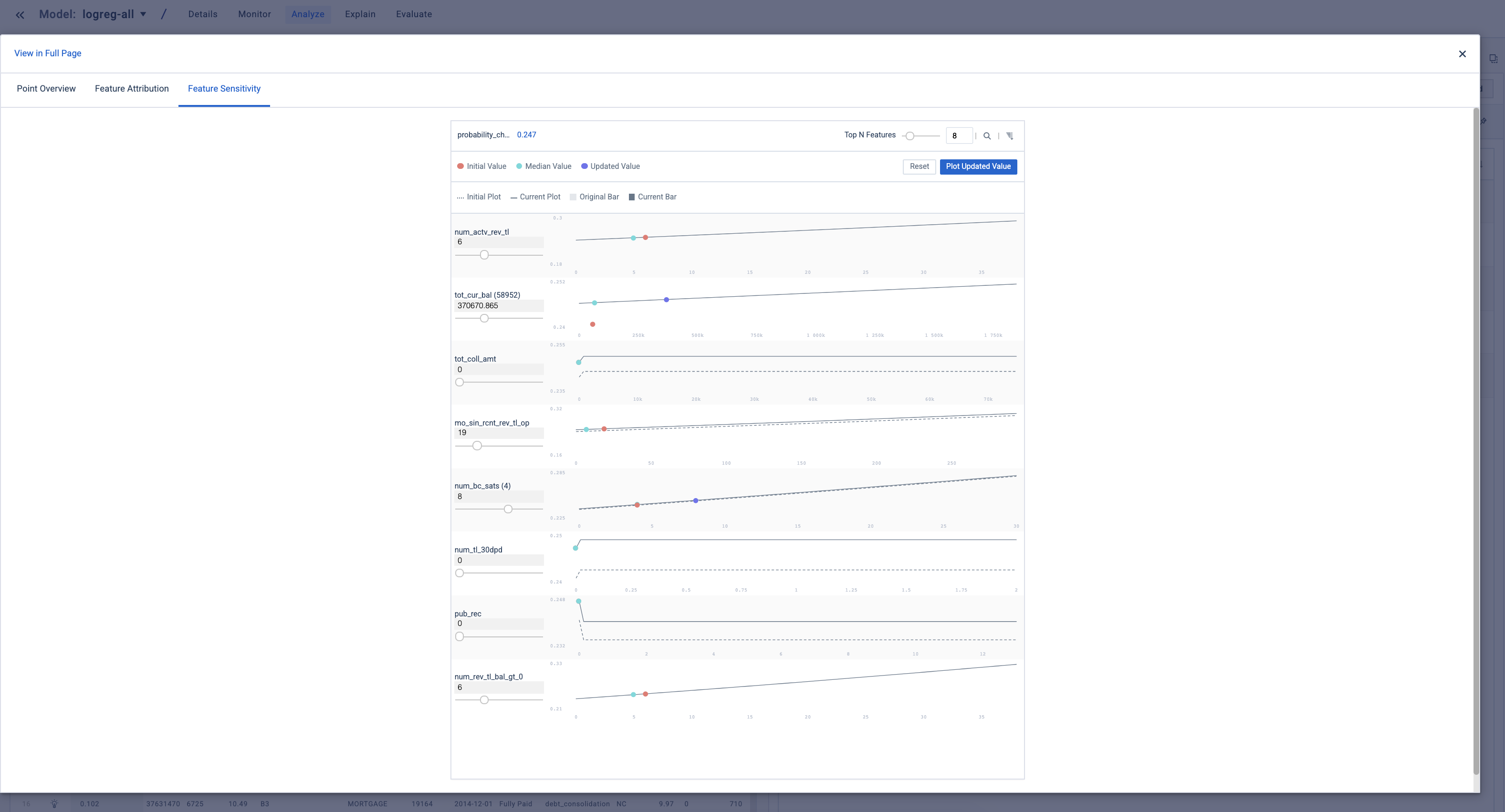## Dashboard

Once visualizations are created, you can pin them to the project dashboard, which can be shared with others.

To pin a chart, click on the thumbtack icon and click Send. If the Update with Query option is enabled, the pinned chart will update automatically whenever the underlying query is changed on the Analyze tab.